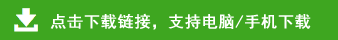# 新人教版小学一年级数学上册综合模拟测试卷（6套）

三、在(    )里填数 (12分)

(   )+7=10    9+7=(   )    10-7=(   )-4

7+(   )=14    8+(   )=16    3+9=(   )+2

9+(   )=14    10-8=(   )    4+8=9+(   )

(   )+9=18    4+9=(   )    (   )+5=17-7

9+2○8    7+6○5+8    5+2+7○10

13○7+8    10+9○10+7    16○9+7

7+7○5+3    10+8○0+9    9-2○2+5